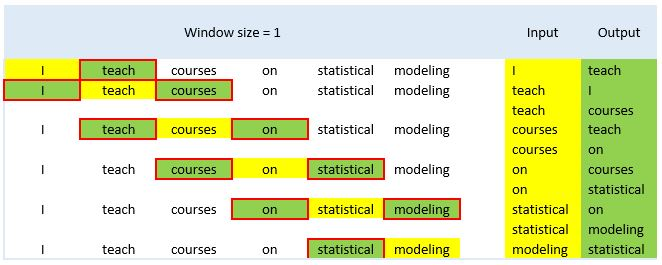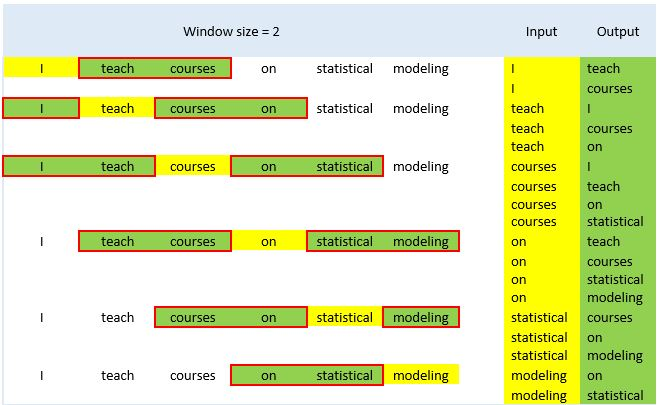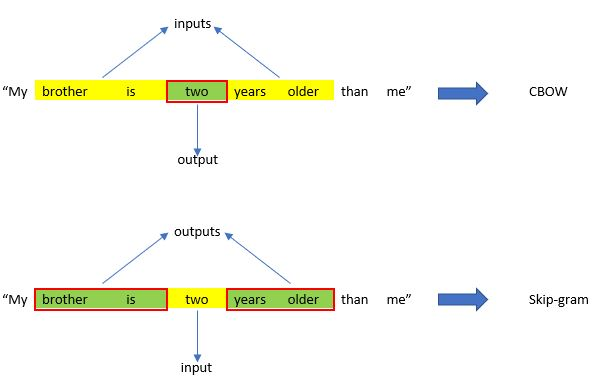# Text Vectorization Using Python: Word2Vec

In the first two part of this series, we demonstrated how to convert text into numerical representation (i.e., text vectorization) using the term-document matrix and term frequency-inverse document frequency (TF-IDF) approaches. In the last part of the series, we focus on a more advanced approach, Word2Vec, that can capture the meaning and association of words within a text. First, we will briefly explain how Word2Vec works and then demonstrate how to use Word2Vec in Python.

Sevilay Kilmen https://akademik.yok.gov.tr/AkademikArama/view/viewAuthor.jsp (Bolu Abant Izzet Baysal University)http://www.ibu.edu.tr , Okan Bulut http://www.okanbulut.com/ (University of Alberta)https://www.ualberta.ca
2022-05-02

## Introduction

Assume that you want to send a text message to a friend using your smartphone. After you typed the first word of your message, “Happy”, which word would the smart keyboard on your phone suggest for the next word? “Christmas” or “birthday”? It is quite likely that the smart keyboard will recommend the word “birthday”, instead of “Christmas”. Now, you have “Happy birthday” in the message. What would be the following words? At this point, it is not hard to guess that the smart keyword will suggest “to” and then “you” to turn the whole sentence to “Happy birthday to you”. But, how could the smart keyboard predict words one by one and help you create this sentence? How does it associate the word with each other? Or more broadly, when you have a Google search, how does Google come up with the most relevant website about a word or a phrase that you typed? To understand this magic, let’s step into the magical world of Word2Vec ✋.

### Word2Vec

As we explained in the last two posts, computers need numerical representations to analyze textual data. This process is called “text vectorization”. So far, we have talked about two text vectorization methods: term-document matrix and term frequency-inverse document frequency (TF-IDF). Both methods are very simple and easy-to-use when it comes to transforming textual data into numerical representations. In the last part of this series, we will focus on a more advanced approach, Word2Vec. Before we dive into what Word2Vec is and how it works, we need to define an important term, word embedding, which refers to an efficient and dense representation where words or phrases with similar meaning are closer in the vector space. In other words, a word embedding refers to a vector representation of a particular word or phrase in a multidimensional space. The vectorization of words or phrases as word embeddings facilitates the estimation of semantic similarities between different text materials (e.g., documents).

There are several word embedding techniques that are widely used in natural language processing (NLP) applications, such as Word2Vec, GloVe, and BERT. In this post, we will talk about Word2Vec developed by Tomas Mikolov and other researchers at Google for semantic analysis tasks. In the Word2Vec method, word vectors (i.e., target word) are constructed based on other words (i.e., context words) that are semantically similar to the target word. The number of context words coming before or after the target word is called “window size”. Now, let’s see two examples where there is a single word before and after the target word (i.e., window size = 1) and there are are two words before and after the target word (i.e., window size = 2):Figure 1: Input and output words based on window size=1Figure 2: Input and output words based on window size=2

Depending on whether context words are used as either input or output, Word2Vec offers two neural network-based variants: Continuous Bag of Word (CBOW) and Skip-gram models. In the CBOW, context words are considered as input and the target word as output, whereas in the Skip-gram architecture, the target word is considered as the input and the context words as the output. The following example shows how input and output words are utilized within the CBOW and Skip-gram models.Figure 3: CBOW and Skipgram models

Let’s assume that the word “two” in the sentence of “My brother is two years older than me” is the target word and the window size is two. In the CBOW model, “two” is considered as output, and the words “brother”, “is”, “years”, and “older” as input. In contrast, in the Skip-gram model, the word “two” is considered as the input, and the other words become the output. To further describe how the Word2Vec algorithm works, we will use real data (i.e., students’ written responses from an automated essay scoring competition) to prepare word embeddings using the Word2Vec algorithm in Python.

## Example

In this example, we will continue using the same Kaggle data set that comes from an automated essay scoring competition funded by the Hewlett Foundation. The data set includes students’ responses to ten different sets of short-answer items and scores assigned by two human raters. The data set is available here as a tab-separated value (TSV) file. The data set contains five variables:

• Id: A unique identifier for each individual student essay
• EssaySet: An id for each set of essays (ranges from 1 to 10)
• Score1: Rater1’s score
• Score2: Rater2’s score
• EssayText: Students’ responses

For our demonstration, we will use student responses in “Essay Set 2” where students are presented with an investigation procedure to test four different polymer plastics for stretchability and data from that investigation. Students are asked to draw a conclusion based on the research data and describe two ways to improve the experimental design and/or validity of the results.

Now, let’s begin our analysis by importing the Essay Set 2 into Python and format the data in such a way that it contains students’ responses under a single column called “response”.

``````# Import required packages
import gensim``````
``````from gensim.models import Word2Vec, KeyedVectors
import pandas as pd

# Import train_rel_2.tsv into Python
with open('train_rel_2.tsv', 'r') as f:
columns = lines.split('\t')
response = []
for line in lines[1:]:
temp = line.split('\t')
if temp == '2':   # Select the Essay Set 2
response.append(temp[-1])  # Select "EssayText" as a corpus
else:
None

# Construct a dataframe ("data") which includes only response column
data = pd.DataFrame(list(zip(response)))
data.columns = ['response']``````

We can go ahead and review this data set.

``print(data)``
``````                                               response
0     Changing the type of grafin would improve the ...
1     Concluding from the students data that plastic...
2     Two ways that the stundent could've improved t...
3     A conclusion I can make from this experiment i...
4     a.One conclusion I can draw is that plastic B ...
...                                                 ...
1273  a) We can conclude that plastic B is the most ...
1274  "a. One conclusion I have fro this data was th...
1275  3. The second trial(12) is not exactly the sam...
1276  A) I have concluded that based on the students...
1277  Plastic type B stretchable most 22mm in T1 & 2...

[1278 rows x 1 columns]``````

The values at the bottom of the output show that the data set consists of 1278 documents and only one column (i.e., response) which we will use as a corpus in this example. To implement the Word2Vec algorithm, we first need to perform tokenization for all the words in this corpus. Since the corpus is pretty large, we will demonstrate the tokenization process using the first response, and then we will apply tokenization to the entire corpus. Now, let’s have a look at the first response more closely.

``data.response``
``"Changing the type of grafin would improve the student's experiment give a better new at those data. ^P Give the names of each type of plastic type used in this experiment. Each plastic should be the same length. ^P My conclusion is plastic type held up a much stronger than all of the different types.\n"``

Using the `simple_preprocess` function from gensim.utils, we will tokenize the response:

``print(gensim.utils.simple_preprocess("Changing the type of grafin would improve the student's experiment give a better new at those data. Give the names of each type of plastic type used in this experiment. Each plastic should be the same length. My conclusion is plastic type held up a much stronger than all of the different types"))``
``['changing', 'the', 'type', 'of', 'grafin', 'would', 'improve', 'the', 'student', 'experiment', 'give', 'better', 'new', 'at', 'those', 'data', 'give', 'the', 'names', 'of', 'each', 'type', 'of', 'plastic', 'type', 'used', 'in', 'this', 'experiment', 'each', 'plastic', 'should', 'be', 'the', 'same', 'length', 'my', 'conclusion', 'is', 'plastic', 'type', 'held', 'up', 'much', 'stronger', 'than', 'all', 'of', 'the', 'different', 'types']``

As the output shows, after tokenization, all the words in the response are separated into smaller units (i.e., tokens). In the following analysis, each word in the corpus will be handled separately in the Word2Vec process. Now, let’s repeat the same procedure for the entire corpus.

``````response_new=data.response.apply(gensim.utils.simple_preprocess)
response_new``````
``````0       [changing, the, type, of, grafin, would, impro...
1       [concluding, from, the, students, data, that, ...
2       [two, ways, that, the, stundent, could, ve, im...
3       [conclusion, can, make, from, this, experiment...
4       [one, conclusion, can, draw, is, that, plastic...
...
1273    [we, can, conclude, that, plastic, is, the, mo...
1274    [one, conclusion, have, fro, this, data, was, ...
1275    [the, second, trial, is, not, exactly, the, sa...
1276    [have, concluded, that, based, on, the, studen...
1277    [plastic, type, stretchable, most, mm, in, mm,...
Name: response, Length: 1278, dtype: object``````

After tokenization is completed for the entire corpus, we can now create a word embedding model using Word2Vec:

``````# Model parameters
model=gensim.models.Word2Vec(window=5, min_count=2, workers=4, sg=0)

# Train the model
model.build_vocab(response_new, progress_per=1000)
model.train(response_new, total_examples=model.corpus_count, epochs=model.epochs)

# Save the trained model``````
``model.save("./responses.model")``

The hyperparameters used in `gensim.models.Word2Vec` are as follows:

• size: The number of dimensions of the embeddings (the default is 100).
• window: The maximum distance between a target word and words around the target word (the default is 5).
• min_count: The minimum count of words to consider when training the model (the default for is 5).
• workers: The number of partitions during training (the default is 3).
• sg: The training algorithm, either 0 for CBOW or 1 for skip gram (the default is 0).

After the training process is complete, we get a vector for each word. Now, let’s take a look at the vectors of a particular from the corpus (e.g., the word “plastic”) to get an idea of what the generated vector looks like.

``model.wv["plastic"]``
``````array([ 0.3236925 ,  0.2548069 ,  0.61545634,  0.1828132 ,  0.31981272,
-0.8854959 ,  0.10059591,  0.5529515 , -0.14545196, -0.33099753,
-0.1684745 , -0.80204433, -0.07991576,  0.10517135,  0.29105937,
-0.08265342,  0.1387488 , -0.44342119, -0.14201172, -1.1230628 ,
0.93303484,  0.15602377,  0.7197224 , -0.35337123, -0.01448521,
0.51030767, -0.06602395,  0.30631196, -0.05907682,  0.11381093,
0.3613567 ,  0.17538303,  0.501223  , -0.46734655, -0.3349126 ,
0.01602843,  0.51649153,  0.22251019, -0.31913355, -0.42772195,
0.05480129,  0.28686902, -0.55821824,  0.20228569, -0.01934895,
-0.4905142 , -0.43356672, -0.40940797,  0.56560874,  0.60450554,
0.10609645, -0.57371974,  0.09981435, -0.48511255,  0.32300022,
0.09809875,  0.11661741,  0.00955052, -0.2510347 ,  0.3500143 ,
-0.27248862,  0.0071716 ,  0.25264668, -0.03935822, -0.13833411,
0.63956493,  0.02967284,  0.48678428, -0.34669146,  0.22514342,
-0.32918864,  0.6453007 ,  0.2724857 ,  0.0536106 ,  0.4775878 ,
0.05614332,  0.5871811 , -0.58996713, -0.26652348, -0.33927533,
-0.6071714 ,  0.29880825, -0.56886315,  0.6028666 , -0.2625632 ,
-0.25126255,  0.6333157 ,  0.3476705 ,  0.28564158, -0.01256744,
1.1550713 ,  0.3539902 ,  0.13358444,  0.30924886,  0.9343885 ,
0.14220482,  0.03595947,  0.12772141, -0.03011671, -0.03848448],
dtype=float32)``````

The vector for the word “plastic” is essentially an array of numbers. Once we convert a word to a numerical vector, we can calculate its semantic similarity with other words in the corpus. For example, let’s find the top 2 words that are semantically the closest to the word “experimental” based on the cosine similarity between the vectors of the words in our corpus.

``model.wv.most_similar("experimental", topn=2)``
``[('improved', 0.9936665892601013), ('design', 0.9927185773849487)]``

We see that the words that are similar to the word “experimental” include “improved” and “design”. We can also calculate the similarity among specific words. In the following example, we will see the similarity of the word “plastic” with two other words, “experiment” and “length”.

``model.wv.similarity(w1="experiment", w2="plastic")``
``0.23159748``
``model.wv.similarity(w1="length", w2="plastic")``
``0.5783539``

The similarity results show that the word “plastic” seems to be closer to the word “length” than the word “experimental”. We must note that this interesting finding is valid only for the corpus we used in this example (i.e., responses from Essay Set 2). The Gensim library in Python also provides pre-trained models and corpora. A pre-trained model based on a massive data set (e.g. the Google News data set) can be used for exploring semantic similarities as long as the data set is relevant to the domain we are working on.

## Conclusion

In this post, we wanted to demonstrate how to use Word2Vec to create word vectors and to calculate semantic similarities between words. Word2Vec transforms individual words or phrases into numerical vectors in a multidimensional semantic space. Word embeddings obtained from Word2Vec can be used for a variety of applications such as generating cloze sentences, query auto-completion, and building a recommendation system. For readers who want to learn more about Word2Vec, we recommend Tensorflow’s Word2Vec tutorial. With this post, we have come to the end of our three-part text vectorization series. We hope that the examples we presented here and in the last two posts help researchers who are interested in using text vectorization for their NLP applications.

### Reuse

Text and figures are licensed under Creative Commons Attribution CC BY 4.0. The figures that have been reused from other sources don't fall under this license and can be recognized by a note in their caption: "Figure from ...".

### Citation

`Kilmen & Bulut (2022, May 2). Okan Bulut: Text Vectorization Using Python: Word2Vec. Retrieved from https://okan.cloud/posts/2022-05-02-text-vectorization-using-python-word2vec/`
```@misc{kilmen2022text,